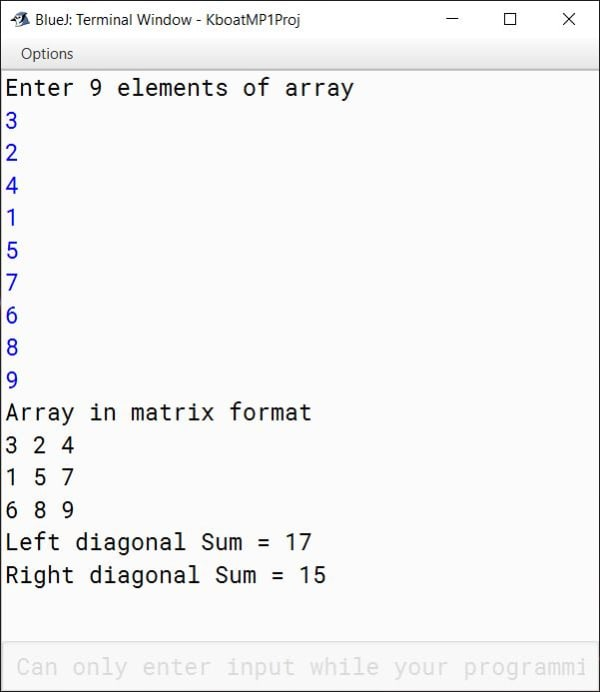# Solved Model Question Paper 1

## Section A

#### Question 1

(a) What is meant by a package? Name any two Java API packages In Java, a package is used to group related classes. Packages are of 2 types:

1. Built-In packages — These are provided by Java API
2. User-Defined packages — These are created by the programmers to efficiently structure their code.
java.util, java.lang are two examples of Java API packages.

(b) What is a wrapper class? Give two examples
Wrapper class wraps the value of primitive type into an object. It provides many utility methods like conversion between primitive type and String and constants like the smallest and largest possible values of the primitive types. Wrapper classes are part of java.lang package. Double and Character are two examples of wrapper classes.

(c) What do you understand by 'Run Time' error? Explain with an example
Errors that occur during the execution of the program primarily due to the state of the program which can only be resolved at runtime are called Run Time errors.
Consider the below example:

```import java.util.Scanner;
class RunTimeError
{
public static void main(String args[]) {
Scanner in = new Scanner(System.in);
System.out.print("Enter a number: ");
int n = in.nextInt();
int result = 100 / n;
System.out.println("Result = " + result);
}
}```

This program will work fine for all non-zero values of n entered by the user. When the user enters zero, a run-time error will occur as the program is trying to perform an illegal mathematical operation of division by 0. When we are compiling the program, we cannot say if division by 0 error will occur or not. It depends entirely depends on the state of the program at run-time.

(d) Differentiate between primitive data types and composite data types

PrimitiveComposite
Primitive Data Types are the fundamental data types provided by Java to store numbers, characters and boolean valuesComposite Data Types are constructed by the programmer by putting together one or more Primitive Data Types
byte, short, int, long, float, double, char, boolean are examples of Primitive Data Typesarray, class and interface are examples of Composite Data Types

(e) What is Polymorphism?

The word Polymorphism means having many forms. In object-oriented programming languages, Polymorphism provides the means to perform a single action in multiple different ways. In Java, it is supported through 2 concepts — Method Overloading and Method Overriding. In both of these concepts, there is a function which shows different behaviours depending on its arguments or the object in the class hierarchy which is implementing it.

#### Question 2

(a) What is the return type of the following library functions:
(i) isWhiteSpace( char )
boolean
(ii) replace(char, char)
String

(b) Predict the output of the below code snippet:

```int a = 345, b = 0;
a--;
b = a == 344 ? 3 : 44;
System.out.println("b = " + b);```
```b = 3
```
##### Explanation

`a--` will decrement a to 344. As the condition of ternary operator is true, it will return 3 which is assigned to b.

(c) Transform the conditional statement of the above question into if else statement

```if (a == 344)
b = 3;
else
b = 44;```

(d) If int x = 5; find the value of the following expressions:
(i) (5 * ++x) % 3
(5 * 6) % 3 = 0
(ii) 'x' + 50
ASCII Value of x + 50
⇒ 120 + 50 = 170

(e) Find the errors and correct them

```int a= 10; b=15;
a = + b;
system.out.print( a);```
##### Corrected Program
```int a= 10, b=15;                //1st Correction
a += b;                     //2nd Correction
System.out.print( a);   //3rd Correction```

(f) If num[] = { 2, 7, 4 , 5, 8};
What is num.length? What is num*4?
num.length = 5, num*4 = 4*4 = 16

#### Question 3

(a) Give the output of the following expressions
(i) If x = 372.25, calculate Math.ceil(x);
373.0
(ii) If x = -4.5, calculate Math.abs(x);
4.5

(b) Given int a=5, Find the value of a after the execution of the statement
`a += a++ + --a + a*2`
a += a++ + --a + a*2
a = a + (a++ + --a + a*2)
a = 5 + (5 + 5 + 5*2)
a = 5 + (5 + 5 + 10)
a = 25

(c) Write a Java function to convert any data type to String data type
String.valueOf

(d) Write a Java function that returns -1 if a searched character is not found
indexOf

(e) If there are many return statements used within a function, how many will be executed and why?
Only one return statement will be executed because the return statement transfers the program control back to the calling method so the remaining return statements will not get executed.

(f) Write down Java expression for √(A2 + B2 + C2)
Math.sqrt(a * a + b * b + c * c);

(g) Write the output of the following program and also tell how many times the loop gets executed:

```for (int a = 5; a <= 30; a += 5)
{
if (a % 3 == 0)
break;
else if (a % 5 == 0)
System.out.println(a);
continue;
}```
##### Output
```5
10
```

Loop is executed 3 times

(h) State the output of the following program segment:

```String s1 = "67420";
String s2 = "character";
System.out.print(s1.substring(2));
System.out.print(" CHA" + s2.substring(0,3).toUpperCase());```
##### Output
```420 CHACHA
```

(i) Convert the following for loop to corresponding while loop

```int n = 5;
for (int a = 10; a >= 1; a--)
System.out.println(a * n);```
##### Converted Loop
```int n = 5, a = 10;
while (a >= 1) {
System.out.println(a * n);
a--;
}```

(j) What will be the output when the following program segment is executed:

`System.out.println("Doctor said \"An apple a day keeps the doctor away\" to me.");`
##### Output
```Doctor said "An apple a day keeps the doctor away" to me.
```

(k) What will be the output when the following program segment is executed:

`System.out.println(Character.isLetter('9'));`
```false
```

## Section B

#### Question 4

Define a class named MovieRating with the following description:

Data MembersPurpose
int yearTo store the year of release of a movie
String titleTo store the title of the movie
float ratingTo store the popularity rating of the movie
Member MethodsPurpose
MovieRating()Default constructor to initialize the data members
void input()To input and store year, title and rating
void display()To display the title of the movie and a message based on the ratings table given below
##### Ratings Table
RatingMessage to be displayed
0.0 to 2.0Flop
2.1 to 3.4Semi-Hit
3.5 to 4.4Hit
4.5 to 5.0Super-Hit

Write a main method to create an object of the class and call the above member methods

```import java.util.Scanner;

public class MovieRating
{
private int year;
private String title;
private float rating;

public MovieRating() {
year = 0;
title = "";
rating = 0.0f;
}

public void input() {
Scanner in = new Scanner(System.in);
System.out.print("Enter Title of Movie: ");
title = in.nextLine();
System.out.print("Enter Year of Movie: ");
year = in.nextInt();
System.out.print("Enter Rating of Movie: ");
rating = in.nextFloat();
}

public void display() {
String message = "Invalid Rating";
if (rating >= 0.0f && rating <= 2.0f)
message = "Flop";
else if (rating <= 3.4f)
message = "Semi-Hit";
else if (rating <= 4.4f)
message = "Hit";
else if (rating <= 5.0f)
message = "Super-Hit";

System.out.println(title);
System.out.println(message);
}

public static void main(String args[]) {
MovieRating obj = new MovieRating();
obj.input();
obj.display();
}
}```
##### Output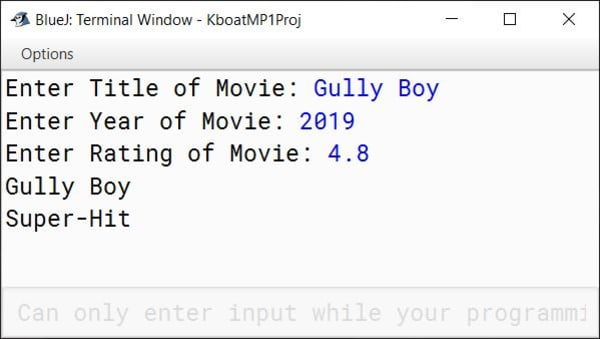#### Question 5

Write a program to accept the year of graduation from school as an integer value from the user. Using the Binary Search technique on the sorted array of integers given below, output the message "Record exists" if the value input is located in the array. If not, output the message "Record does not exist".
{1982, 1987, 1993. 1996, 1999, 2003, 2006, 2007, 2009, 2010}

```import java.util.Scanner;

{
Scanner in = new Scanner(System.in);
int n[] = {1982, 1987, 1993, 1996, 1999, 2003, 2006, 2007, 2009, 2010};

System.out.print("Enter graduation year to search: ");
int year = in.nextInt();

int l = 0, h = n.length - 1, idx = -1;
while (l <= h) {
int m = (l + h) / 2;
if (n[m] == year) {
idx = m;
break;
}
else if (n[m] < year) {
l = m + 1;
}
else {
h = m - 1;
}
}

if (idx == -1)
System.out.println("Record does not exist");
else
System.out.println("Record exists");
}
}```
##### Output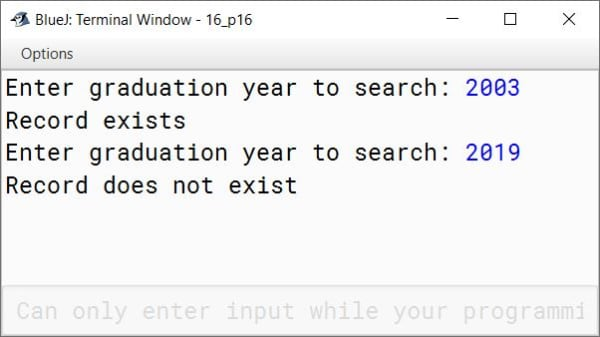#### Question 6

Write a program to accept a number from the user and check whether it is a Neon number or not. A Neon number is a number where the sum of digits of square of the number is equal to the number.
Example:
Consider the number 9
Square of 9 = 9 x 9 = 81
Sum of the digits of the square = 8 + 1 = 9
Hence 9 is a Neon Number.

```import java.util.Scanner;

public class NeonNumber
{
public static void main(String args[]) {
Scanner in = new Scanner(System.in);
System.out.print("Enter the number to check: ");
int n = in.nextInt();
int sq = n * n;
int sqSum = 0;

while (sq != 0) {
int d = sq % 10;
sqSum += d;
sq /= 10;
}

if (sqSum == n)
System.out.println("Neon Number");
else
System.out.println("Not a Neon Number");
}
}```
##### Output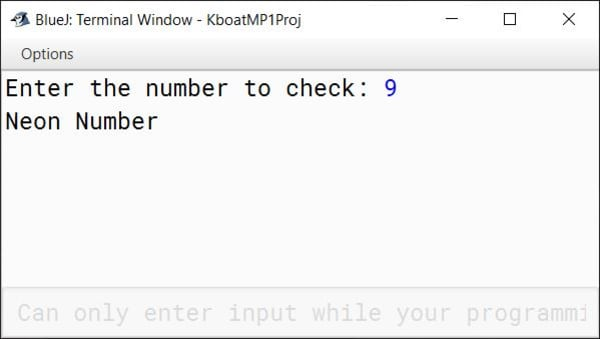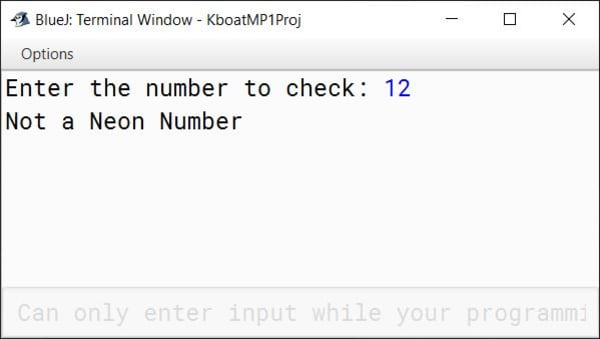#### Question 7

Using switch statement, write a menu driven program to display the pattern as per user's choice:

Pattern 1
4321
321
21
1

Pattern 2
@
&&
@@@
&&&&
@@@@@

```import java.util.Scanner;

public class KboatUserChoicePattern
{
public static void main(String args[]) {
Scanner in = new Scanner(System.in);
System.out.println("Type 1 for Pattern 1");
System.out.println("Type 2 for Pattern 2");
int ch = in.nextInt();

switch (ch) {
case 1:
for (int i = 4; i >= 1; i--) {
for (int j = i; j >= 1; j--) {
System.out.print(j);
}
System.out.println();
}
break;

case 2:
for (int i = 1; i <= 5; i++) {
char t = i % 2 == 0 ? '&' : '@';
for (int j = 1; j <= i; j++) {
System.out.print(t);
}
System.out.println();
}
break;

default:
System.out.println("Invalid Choice");
break;
}
}
}```
##### Output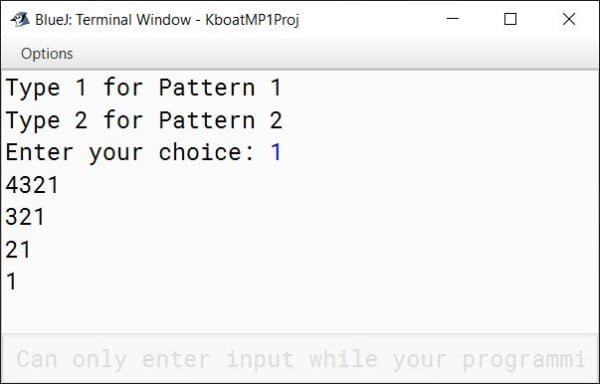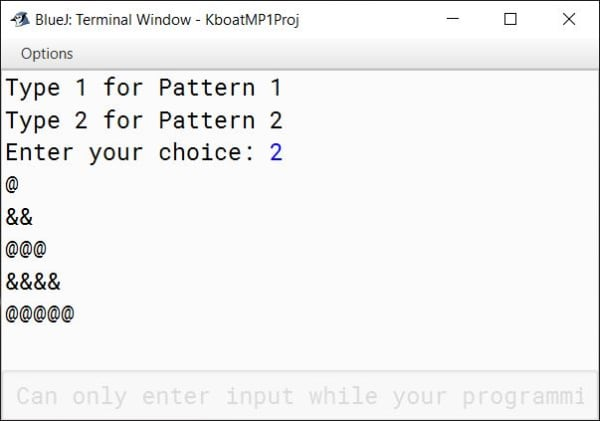#### Question 8

Design a class named Tom to overload a function TalkingTom() as follows:

1. void TalkingTom(String s1, String s2) with two string arguments and print the string which is greater in length.
2. void TalkingTom(String s) with one string argument and print the first and the last character of the string.
3. int TalkingTom(String s, char ch) with one string argument and one character argument and return the frequency of the character argument in the given string

Write main method to call above member methods to display the output.

```public class Tom
{
public void TalkingTom(String s1, String s2) {
if (s1.length() > s2.length())
System.out.println(s1);
else
System.out.println(s2);
}

public void TalkingTom(String s) {
System.out.println("First Character = " + s.charAt(0));
System.out.println("Last Character = " +
s.charAt(s.length() - 1));
}

public int TalkingTom(String s, char ch) {
int freq = 0;
for (int i = 0; i < s.length(); i++) {
if (s.charAt(i) == ch)
freq++;
}

return freq;
}

public static void main(String args[]) {
Tom obj = new Tom();
obj.TalkingTom("small", "big");
obj.TalkingTom("KnowledgeBoat");
int a = obj.TalkingTom("KnowledgeBoat", 'o');
System.out.println("Frequency of o in KnowledgeBoat = "
+ a);
}
}```
##### Output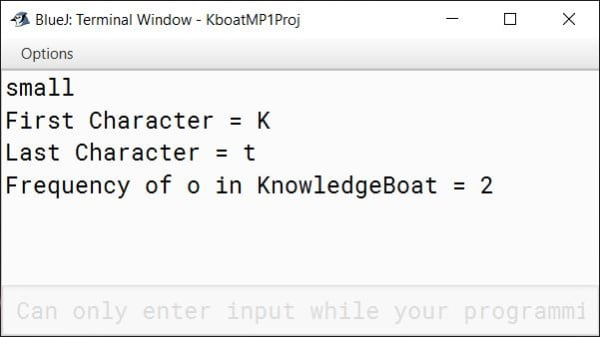#### Question 9

Write a program to input and store integer elements in a double dimensional array of size 3x3 and perform the following tasks:

1. Display the elements of the 3x3 array in matrix format
2. Find the sum of elements in the left diagonal
3. Find the sum of elements in the right diagonal

Example:
3x3 Array = {{3, 2, 4}, {1, 5, 7}, {6, 8, 9}}
Array in matrix format:
3 2 4
1 5 7
6 8 9
Sum of elements in the left diagonal = 17
Sum of elements in the right diagonal = 15

```import java.util.Scanner;

{
public static void main(String args[]) {
Scanner in = new Scanner(System.in);
int arr[][] = new int;
System.out.println("Enter 9 elements of array");
for (int i = 0; i < 3; i++) {
for (int j = 0; j < 3; j++)
arr[i][j] = in.nextInt();
}

int lSum = 0, rSum = 0;
System.out.println("Array in matrix format");
for (int i = 0; i < 3; i++) {
for (int j = 0; j < 3; j++) {
System.out.print(arr[i][j] + " ");
if (i == j)
lSum += arr[i][j];
if (i + j == 2)
rSum += arr[i][j];
}
System.out.println();
}

System.out.println("Left diagonal Sum = " + lSum);
System.out.println("Right diagonal Sum = " + rSum);
}
}```
##### Output